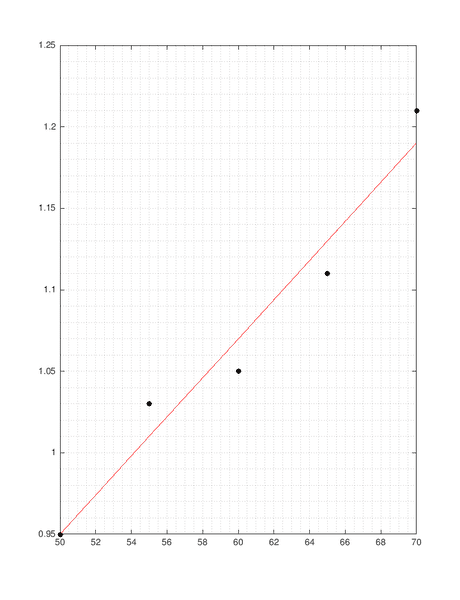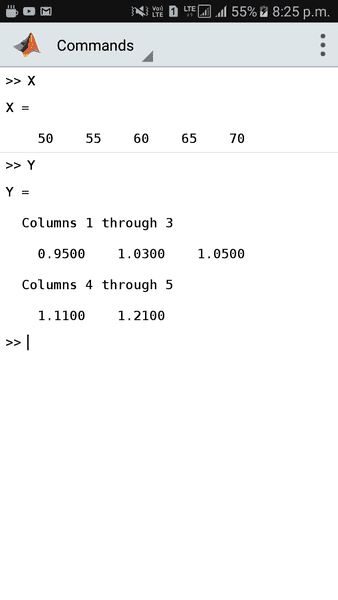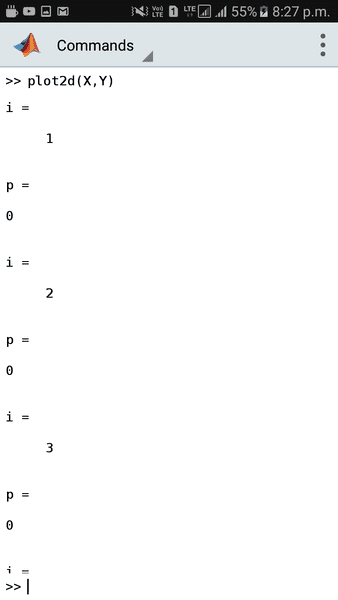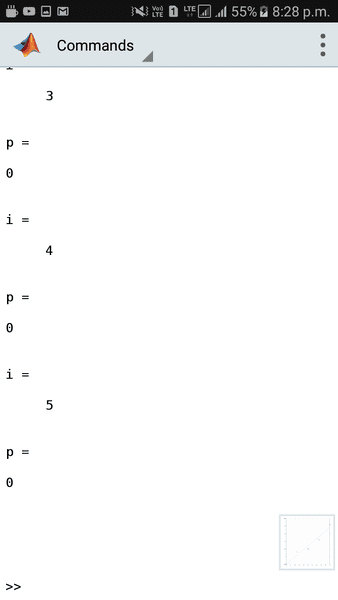# Best fit line for data points

Gold Member
I have some experimental data, and I would like to plot a graph in matlab and also find the best fit line. I also want to find the distance between each point and the best fit line, and if the distance is greater than 1 unit, an error will be shown.

For this, I have written a function. Here it is:

Matlab:
function [] = plot2d(X,Y);

%The aim of this function is to read
% X and Y from the user, and plot the points.
% The best fit line shall be shown.

hold off;

%this plots the given data points
plot(X,Y,'.k', 'MarkerSize', 20);

hold on;

%here, we are calculating the best fit line
p = polyfit(X,Y,1);
f = polyval(p, X);
plot(X, f, '-r');

%necessary plot settings
zoom on;
set(gca, 'xminorgrid', 'on', 'yminorgrid', 'on');

syms x1 y1 x2 y2 x y;

%finding the equation of the best fit line
x1 = X(1);
x2 = X(end);
y1 = f(1);
y2 = f(end);
m = (y2 - y1) / (x2 - x1);

eqn = y - y1 == m * (x - x1);

%simplifying the equation
eqn = simplify(eqn);

%writing the equation in Ax + By + C = 0 form and removing the rhs to make it an algebraic expression
eqn2 = lhs(eqn) - rhs(eqn);

coefx = coeffs(eqn2,x);

%coefficient of x
cx = coefx(end);

coefy = coeffs(eqn2,y);

%coefficient of y
cy = coefy(end);

%finding number of data points given
sz = numel(X);

for i = 1:1:sz

x = X(i);
y = f(i);

%evaluating the expression by putting in values
p = subs(eqn2);

%calculating final distance
dist = p / sqrt(cx^2 + cy^2);

if dist > 1
disp("Error for point (" + x + "," + y + ")");
end

end

hold off;

end
Matlab gives me a good graph:The data is:But all distances of points are coming to be 0. Because the subs(eqn2) is yielding a 0. From the graph, it is evident that all points do not lie on the line. But I'm still getting a 0. I know this because I printed p from the function. This is what I got:Any help is appreciated. Where am I going wrong?

Any ideas?

I use matlab mobile, version R2018a.

Last edited:

## Answers and Replies

Mentor
My suggestion is to make some test data and a small program that illustrates the problem. Focus on the subs and at the Matlab api description of subs to see if you’re using it correctly.

Usually there are examples of the function that you can try too.

Gold Member
My suggestion is to make some test data and a small program that illustrates the problem. Focus on the subs and at the Matlab api description of subs to see if you’re using it correctly.

Usually there are examples of the function that you can try too.
The problem is that, I tried by putting values in eqn2 myself. I did this by printing eqn2, and then manually putting in values. The answers are truly coming to 0. But from the graph, the distance cannot be 0. That is the bottleneck. The points do not lie on the best fit line, but the equation of the best fit line shows that all the points lie on it. I think the problem is coming from the polyfit or polyval functions.

Mentor
What is this line used for?

Matlab:
    eqn = y - y1 == m * (x - x1);

Gold Member
What is this line used for?

Matlab:
    eqn = y - y1 == m * (x - x1);
That line finds the equation of the best fit line using the formula $$y - y_1 = m(x-x_1)$$

Gold Member
Is your "distance" the Y error or is it the perpondicular distance to the line? If it is the former, there are easier ways to get the Y error. If it is the latter, consider principle components.

Gold Member
Is your "distance" the Y error or is it the perpondicular distance to the line? If it is the former, there are easier ways to get the Y error. If it is the latter, consider principle components.
It is the perpendicular distance of each point from the best fit line.

Can you elaborate a bit on the principle components? For computation of distance, I have used the formula $$\dfrac{Ax+By+C}{\sqrt{A^2+B^2}}$$ where ##x## and ##y## are from the data.

Gold Member
The MATLAB function polyfit is not doing what you want to do. It is minimizing the sum-squares of the y errors -- the vertical distance to the line.
To minimize the sum-squared perpendicular distances, the first principle component is what you want. From https://en.wikipedia.org/wiki/Principal_component_analysis#Further_considerations :

"Given a set of points in Euclidean space, the first principal component corresponds to a line that passes through the multidimensional mean and minimizes the sum of squares of the distances of the points from the line."

Gold Member
The MATLAB function polyfit is not doing what you want to do. It is minimizing the sum-squares of the y errors -- the vertical distance to the line.
To minimize the sum-squared perpendicular distances, the first principle component is what you want. From https://en.wikipedia.org/wiki/Principal_component_analysis#Further_considerations :

"Given a set of points in Euclidean space, the first principal component corresponds to a line that passes through the multidimensional mean and minimizes the sum of squares of the distances of the points from the line."
Two questions:

1. What is the difference between vertical and perpendicular distances?
2. Is there any known function in matlab to make a graph from first PCA?

Gold Member
1) See, for instance, https://benediktehinger.de/blog/sci...sion-lines-and-the-first-principal-component/
2) In MATLAB, use [COEFF,SCORE] = princomp(X). The first row of COEFF will give you the first principle component. I think that you will have to plot it yourself.
Thanks for the function. But princomp has been removed; it has now become pca in R2018a.

However, I will not be able to use it, because it requires me to buy the Statistics toolbox. In fact, my matlab is not licensed, and that's why I can use it only on mobile.Gold Member
A good, free statistics package is R. It is well respected and documented. In fact, it ranks fairly high on the list of most often used programming languages. But naturally there is a learning curve. If you will do a lot of statistics, it may be worth getting and learning.

Apparently, PCA can be done in Excel. I am not familiar with that. See https://www.quora.com/Which-is-the-...xcel-to-perform-principal-components-analysis

Last edited:
•Wrichik Basu
Gold Member
I am indeed heartbroken with matlab. Just now, I found that they have stopped supporting 32-bit pc. The last supporting version was R2015b, which is no longer sold now. Perhaps I am done with matlab for the moment, and I should shift to some other language.

Image Analyst
Or perhaps you should upgrade your computer.

Gold Member
Or perhaps you should upgrade your computer.
That's costly. What I have decided is, I will buy the software and additional toolboxes, but keep on using it in the mobile app. I don't need simulink at this moment, so I think I'm fine with whatever I can do on the app.